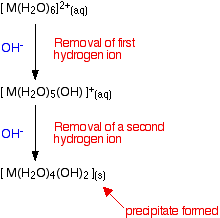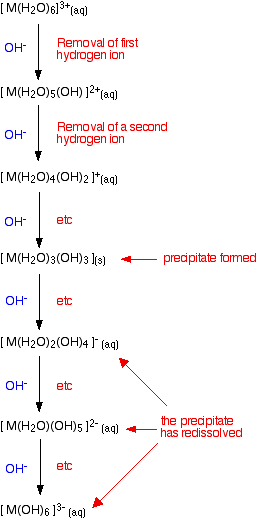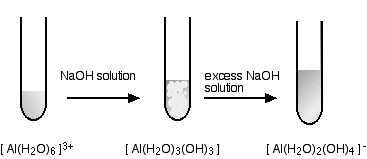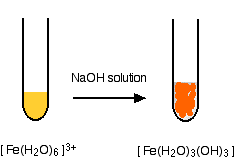# Reactions of the Hexaaqua Ions with Hydroxide Ions

$$\newcommand{\vecs}{\overset { \rightharpoonup} {\mathbf{#1}} }$$ $$\newcommand{\vecd}{\overset{-\!-\!\rightharpoonup}{\vphantom{a}\smash {#1}}}$$$$\newcommand{\id}{\mathrm{id}}$$ $$\newcommand{\Span}{\mathrm{span}}$$ $$\newcommand{\kernel}{\mathrm{null}\,}$$ $$\newcommand{\range}{\mathrm{range}\,}$$ $$\newcommand{\RealPart}{\mathrm{Re}}$$ $$\newcommand{\ImaginaryPart}{\mathrm{Im}}$$ $$\newcommand{\Argument}{\mathrm{Arg}}$$ $$\newcommand{\norm}{\| #1 \|}$$ $$\newcommand{\inner}{\langle #1, #2 \rangle}$$ $$\newcommand{\Span}{\mathrm{span}}$$ $$\newcommand{\id}{\mathrm{id}}$$ $$\newcommand{\Span}{\mathrm{span}}$$ $$\newcommand{\kernel}{\mathrm{null}\,}$$ $$\newcommand{\range}{\mathrm{range}\,}$$ $$\newcommand{\RealPart}{\mathrm{Re}}$$ $$\newcommand{\ImaginaryPart}{\mathrm{Im}}$$ $$\newcommand{\Argument}{\mathrm{Arg}}$$ $$\newcommand{\norm}{\| #1 \|}$$ $$\newcommand{\inner}{\langle #1, #2 \rangle}$$ $$\newcommand{\Span}{\mathrm{span}}$$$$\newcommand{\AA}{\unicode[.8,0]{x212B}}$$

This page describes and explains the reactions between complex ions of the type [M(H2O)6]n+ and hydroxide ions from, for example, sodium hydroxide solution. It assumes that you know why these ions are acidic, and are happy about the equilibria involved.

## The general case

Although there are only minor differences, for simplicity we will look at 2+ ions and 3+ ions separately.

### Adding hydroxide ions to 2+ hexaaqua ions

These have the form [M(H2O)6]2+. Their acidity is shown in the reaction of the hexaaqua ions with water molecules from the solution:

$\ce{[M(H_2O)_6]^{2+} (aq) + H2O (l) \rightleftharpoons [M(H_2O)_5(OH)]^{+} (aq) + H3O^+ (aq)} \nonumber$

They are acting as acids by donating hydrogen ions to water molecules in the solution. Because of the confusing presence of water from two different sources (the ligands and the solution), it is easier to simplify this:

$\ce{[M(H_2O)_6]^{2+} (aq) \rightleftharpoons [M(H_2O)_5(OH)]^{+} (aq) + H^+ (aq)} \nonumber$

### Disturbing this equilibrium by adding hydroxide ions - stage 1

What happens if you add hydroxide ions to this equilibrium? There are two possible reactions.

1. Reaction of hydroxide ions with the hydroxonium ions (hydrogen ions). According to Le Chatelier's Principle, the position of equilibrium will move to the right, producing more of the new complex ion.1. Reaction of hydroxide ions with the hexaaqua ion. Statistically, there is far more chance of a hydroxide ion hitting a hexaaqua metal ion than of hitting a hydrogen ion. There are far more hexaaqua ions present. If that happens, you get exactly the same new complex ion formed as above. $\ce{[M(H_2O)_6]^{2+} (aq) + OH^{-} (aq) \rightleftharpoons [M(H_2O)_5(OH)]^{+} (aq) + H2O (l)} \nonumber$

Notice that this isn't a ligand exchange reaction. The hydroxide ion has removed a hydrogen ion from one of the ligand water molecules. The reaction has also become essentially irreversible.

### The second stage of the reaction

Whichever of the above reactions happens, you end up with [M(H2O)5(OH)]+ ions in solution. These are also acidic, and can lose hydrogen ions from another of the water ligands. Taking the easier version of the equilibrium:

$\ce{ [M(H_2O)_5(OH)]^{+} (aq) \rightleftharpoons [M(H_2O)_4(OH)_2](s) + H^{+} (aq) } \nonumber$

Adding hydroxide ions again tips the equilibrium to the right - either by reacting with the hydrogen ions, or by reacting directly with the complex on the left-hand side. When this occurs, the new complex formed no longer has a charge - we describe it as a "neutral complex". In all the cases we are looking at, this neutral complex is insoluble in water - and so a precipitate is formed. This precipitate is often written without including the remaining water ligands. In other words we write it as M(OH)2. A precipitate of the metal hydroxide has been formed.

### Summarizing what has happened so far## Going further

There is no logical reason why the removal of hydrogen ions from the complex should stop at this point. Further hydrogen ions can be removed by hydroxide ions to produce anionic complexes - complexes carrying negative charges. Whether this actually happens in the test tube to any extent varies from metal to metal.In fact, if you do this using sodium hydroxide solution of the usual concentrations, most of the 2+ ions that you will meet at this level don't go beyond the precipitate. The only one you are likely to come across is the zinc case - and that has a complication. The final ion is [Zn(OH)4]2- - a tetrahedral ion which has lost the remaining 2 water ligands.

### Adding hydroxide ions to 3+ hexaaqua ions

The argument here is exactly as before - the only difference is the number of hydrogen ions which have to be removed from the original hexaaqua complex to produce the neutral complex. Going beyond the neutral complex is also rather more common with 3+ than with 2+ ions, and may go as far as having a hydrogen ion removed from each of the six water molecules. This is summarized in the same sort of flow scheme as before:## Looking at the ions of specific metals

In each case the formula of the precipitate will be given as if it were the simple neutral complex. In fact, these "hydroxide" precipitates sometimes rearrange by losing water from combinations of the attached OH groups. This produces oxides closely associated with the lost water. These changes are beyond the scope of this site.

## 2+ ions

### hexaaquacobalt(II)### hexaaquacopper(II)### hexaaquairon(II)Iron is very easily oxidized under alkaline conditions. Oxygen in the air oxidizes the iron(II) hydroxide precipitate to iron(III) hydroxide especially around the top of the tube. The darkening of the precipitate comes from the same effect.

### hexaaquamanganese(II)I have shown the original solution as very pale pink (the palest I can produce!), but in fact it is virtually colourless. The pale brown precipitate is oxidized to darker brown manganese(III) oxide in contact with oxygen from the air.

### hexaaquanickel(II)### hexaaquazincYou start and finish with colorless solutions, producing a white precipitate on the way.

## 3+ ions

### hexaaquaaluminiumThis looks exactly the same in the test tube as the corresponding zinc reaction above - but beware the different formulae of the precipitate and the final solution.

### hexaaquachromium(III)### hexaaquairon(III)## Reversing the process

If adding hydroxide ions removes hydrogen ions from the hexaaqua complex one at a time, it doesn't seem unreasonable that you could put them back again by adding an acid. That's just what happens!

We'll look in detail at what happens in the chromium(III) case, but exactly the same principle applies to all the other examples we've looked at - whether for 2+ or 3+ ions. As long as you understand what is happening, you can work out the details if you need to

### Adding acid to hexahydroxochromate(III) ions

These are the ions formed at the end of the sequence in which you add hydroxide ions to a solution containing hexaaquachromium(III) ions. Their formula is [Cr(OH)6]3-. A reminder of the color changes when you add sodium hydroxide solution to a solution containing hexaaquachromium(III) ions:If you add an acid (dilute sulphuric acid, for example), the hydrogen ions get put back on one at a time.You already know the colors of the significant stages (the beginning, the end, and the neutral complex). It isn't a separate bit of learning! You can apply this to any case. If you know the colors as you remove hydrogen ions, you automatically know them as you put the hydrogen ions back on again. It also doesn't matter where you start from either - whether, for example, you add acid to an ionic complex like [Cr(OH)6]3-, or a neutral one like [Fe(H2O)4(OH)2].

You will know that the [Fe(H2O)4(OH)2] is a dirty green precipitate. When you add the hydrogen ions back to it, it will revert to the very pale green solution of the [Fe(H2O)6]2+ ion. None of this is a new bit of learning - you just have to re-arrange what you already know!

## Amphoteric hydroxides

An amphoteric substance has both acidic and basic properties. In other words, it will react with both bases and acids. Some of the metal hydroxides we've been looking at are doing exactly that.

### Chromium(III) hydroxide as an amphoteric hydroxide

"Chromium(III) hydroxide" is a simple way of naming the neutral complex [Cr(H2O)3(OH)3]. You have seen that it reacts with bases (hydroxide ions) to give [Cr(OH)6]3-. It also reacts with acids (hydrogen ions) to give [Cr(H2O)6]3+. This is a good example of amphoteric behavior.

Other examples of amphoteric hydroxides are zinc hydroxide and aluminium hydroxide.

### Copper(II) hydroxide as a basic oxide

Quite a lot of metal hydroxides won't react any further with hydroxide ions if you use sodium hydroxide solution at the sort of concentrations normally used in the lab. That means that they don't have any significant acidic nature.

"Copper(II) hydroxide" is what we would normally call the neutral complex [Cu(H2O)4(OH)2]. This doesn't dissolve in sodium hydroxide solution at any concentration normally used in the lab. It doesn't show any acidic character.

On the other hand, it will react with acids - replacing the lost hydrogen ions on the water ligands. Because it is accepting hydrogen ions, it is acting as a base. Hydroxides like this (which react with acids, but not bases) are not amphoteric - they are just simple bases.